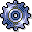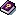Variance AnalysisUse

You can use product drilldown to display the existing variances between planned and actual costs, or target and actual costs (see also :Terminology in Product Cost Controlling)

Variances are the difference between planned and actual costs.

Planned values are adjusted to a reference base, as a rule to the actual values, in order to calculate variances between target and actual costs. This adjustment is performed during variance calculation. You calculate variances in period-end closing of Product Cost by Period or Product Cost by Order. During variance calculation, the variances to be analyzed are distributed to individual variance categories. These variance categories can be displayed in the information system.

If variances have not yet been calculated, target costs can be calculated dynamically in the information system. The target costs are calculated on the basis of a target cost version.

Prerequisites

Before you use the reports Target/Actual/Production Variances and Variance Categories, you must have already performed variance calculation in period-end closing of Product Cost by Order or Product Cost by Period.